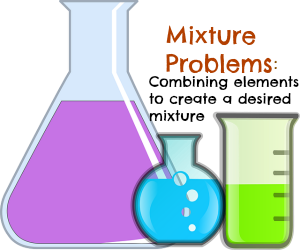# Practicing Mixture Problems in Algebra

Instructor: Laura Pennington

Laura received her Master's degree in Pure Mathematics from Michigan State University, and her Bachelor's degree in Mathematics from Grand Valley State University. She has 20 years of experience teaching collegiate mathematics at various institutions.

Mixture problems in algebra become easier the more we practice them. This lesson will provide multiple practice problems and familiarize us with the general solving process of these types of problems.

## Definition & Steps

Mixture problems in algebra involve combining things such as solutions or objects together to create a desired blend. Although there are multiple types of mixture problems in algebra, they all follow the same basic solving process. In this lesson, we will practice by solving problems that involve adding or taking away an element from a solution to obtain a new solution as well as mixing two solutions together to obtain a new solution.Solving these types of problems takes on the general pattern of the following four steps.

1.) Use a variable to represent the unknown quantity in the problem.

2.) Use the information given in the problem to create an equation involving that variable.

3.) Use algebra to solve the equation for the variable.

4.) Answer the question posed in the problem.

We want to look at multiple examples to practice solving mixture problems. In each of the following practice problems, we will use the four steps listed above as a guide to help us solve the problem.

## Practice Problem #1

Suppose you have a jug of 120 ounces of juice that is 20% pure apple juice and the rest is water. You feel this is a bit too sweet, so you want to add water to the juice so that your new juice is 15% pure apple juice. How much water should you add?

Solution:

1.) Your unknown quantity is how much water you should add to your juice. We will represent this with the variable w.

2.) We want to set up an equation in w with the information given.

The initial jug of juice is 120 ounces, and it contains 20% pure apple juice. We can figure out how many ounces of pure apple juice are in the jug by finding 20% of 120 ounces. We convert 20% to decimal form by multiplying by 1 / 100 to get 0.20. Then we multiply 120 by 0.20. Thus, we have 120 * 0.20 = 24 ounces.

We are adding w ounces of water to our initial juice, so our new juice will contain 120 + w ounces, and 24 of those ounces will still be pure apple juice. We want this new juice to be 15% pure apple juice, so we want 24 to be 15% of 120 + w. Putting this into an equation, we have 0.15( 120 + w ) = 24. This is our equation.

3.) Now we will solve our equation using algebra.4.) We see that w = 40 ounces, so we should add 40 ounces of water to our jug of juice in order to have a juice that is 15% pure apple juice.

## Practice Problem #2

Assume you have 15 gallons of a 15% oil solution. How many gallons of a 30% oil solution need to be added to make a 25% oil solution?

Solution:

1.) You're unknown quantity is how many gallons of the 30% oil solution you should add to your 15% oil solution. We will represent this with the variable s.

2.) We want to set up an equation in s with the information given.

Our initial solution is 15 gallons of 15% oil solution. To find the number of gallons of oil our initial solution contains, we convert 15% to decimal form (as previously demonstrated) to get 0.15. Then we multiply by the 15 gallons. Our initial solution contains 15 * 0.15 = 2.25 gallons of oil. The solution we are adding is s gallons of 30% oil solution, so this solution contains 0.30s gallons of oil.

When we mix these two solutions together, we have s + 15 gallons of mixture, and we want 25% of that to be oil, so we want our mixture to contain 0.25( s + 15) gallons of oil.

Putting this all together to create an equation, we recognize that the amount of oil in the 15% solution (2.25 gallons) plus the amount of oil in the added 30% solution (0.30s gallons) will be equal to the amount of oil in the desired final mixture (0.25(s +15) gallons). In equation form, we have 2.25 + 0.30s = 0.25( s + 15).

3.) Now we solve the equation we found in step 2 using algebra.To unlock this lesson you must be a Study.com Member.

### Register to view this lesson

Are you a student or a teacher?